# Hellinger integral

(diff) ← Older revision | Latest revision (diff) | Newer revision → (diff)

An integral of Riemann type of a set function. If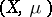is a space with a finite, non-negative, non-singular measure; if,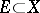, is a totally-additive function with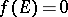for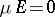; and if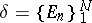is a partition of, then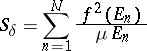and the Hellinger integral of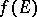with respect tois defined as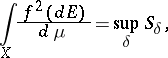provided that this supremum is finite. Hellinger's integral can also be regarded as the limit over a directed set of partitions: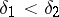if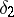is a subdivision of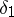.

Ifis a summable function such thatis the Lebesgue integral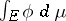, then the Hellinger integral can be expressed in terms of the Lebesgue integral:E. Hellinger in  defined the integral for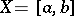in terms of point functions.

How to Cite This Entry:
Hellinger integral. Encyclopedia of Mathematics. URL: http://encyclopediaofmath.org/index.php?title=Hellinger_integral&oldid=18237
This article was adapted from an original article by I.A. Vinogradova (originator), which appeared in Encyclopedia of Mathematics - ISBN 1402006098. See original article